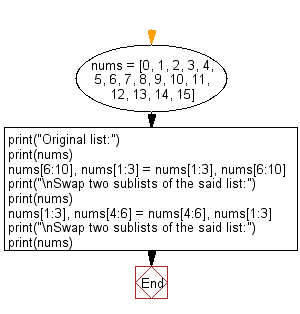﻿ Python: Swap two sublists in a given list - w3resource# Python: Swap two sublists in a given list

## Python List: Exercise - 186 with Solution

Write a Python program to swap two sublists in a given list.

Sample Solution:

Python Code:

``````nums = [0, 1, 2, 3, 4, 5, 6, 7, 8, 9, 10, 11, 12, 13, 14, 15]
print("Original list:")
print(nums)
nums[6:10], nums[1:3] = nums[1:3], nums[6:10]
print("\nSwap two sublists of the said list:")
print(nums)
nums[1:3], nums[4:6] = nums[4:6], nums[1:3]
print("\nSwap two sublists of the said list:")
print(nums)
```
```

Sample Output:

```Original list:
[0, 1, 2, 3, 4, 5, 6, 7, 8, 9, 10, 11, 12, 13, 14, 15]

Swap two sublists of the said list:
[0, 6, 7, 8, 9, 3, 4, 5, 1, 2, 10, 11, 12, 13, 14, 15]

Swap two sublists of the said list:
[0, 9, 3, 8, 6, 7, 4, 5, 1, 2, 10, 11, 12, 13, 14, 15]
```

Flowchart:## Visualize Python code execution:

The following tool visualize what the computer is doing step-by-step as it executes the said program:

Python Code Editor:

Have another way to solve this solution? Contribute your code (and comments) through Disqus.

What is the difficulty level of this exercise?

Test your Python skills with w3resource's quiz

﻿

## Python: Tips of the Day

Floor Division:

When we speak of division we normally mean (/) float division operator, this will give a precise result in float format with decimals.

For a rounded integer result there is (//) floor division operator in Python. Floor division will only give integer results that are round numbers.

```print(1000 // 300)
print(1000 / 300)```

Output:

```3
3.3333333333333335```